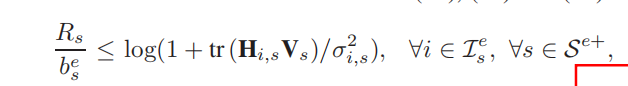# The problem is :Disciplined convex programming error: Cannot perform the operation: {positive constant} ./ {convex}the convex variable is “be”,how can I translate the fomulation to convex?
here is my code
for i=1:se
Rs(i)/inv_pos(be)<=(log(1+trace(He{i}.*V{i})/Noise)/log(2));
end

I think you want
`Rs(i)*inv_pos(be)`

thank you very much .